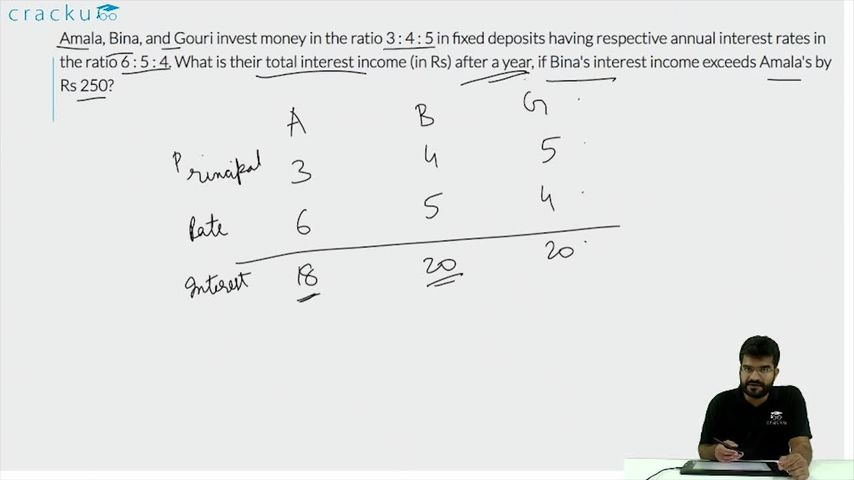Question 77

# Amala, Bina, and Gouri invest money in the ratio 3 : 4 : 5 in fixed deposits having respective annual interest rates in the ratio 6 : 5 : 4. What is their total interest income (in Rs) after a year, if Bina's interest income exceeds Amala's by Rs 250?

Solution

Assuming the investment of Amala, Bina, and Gouri be 300x, 400x and 500x, hence the interest incomes will be 300x*6/100=18x, 400x*5/100=20x and 500x*4/100 = 20x

Given, Bina's interest income exceeds Amala by 20x-18x=2x=250  => x=125

Now, total interest income = 18x+20x+20x=58x = 58*125 = 7250

### View Video Solution